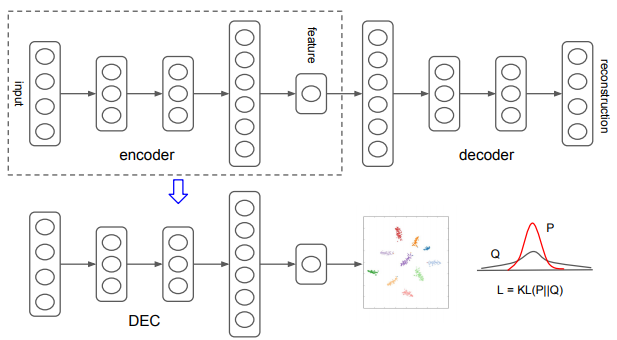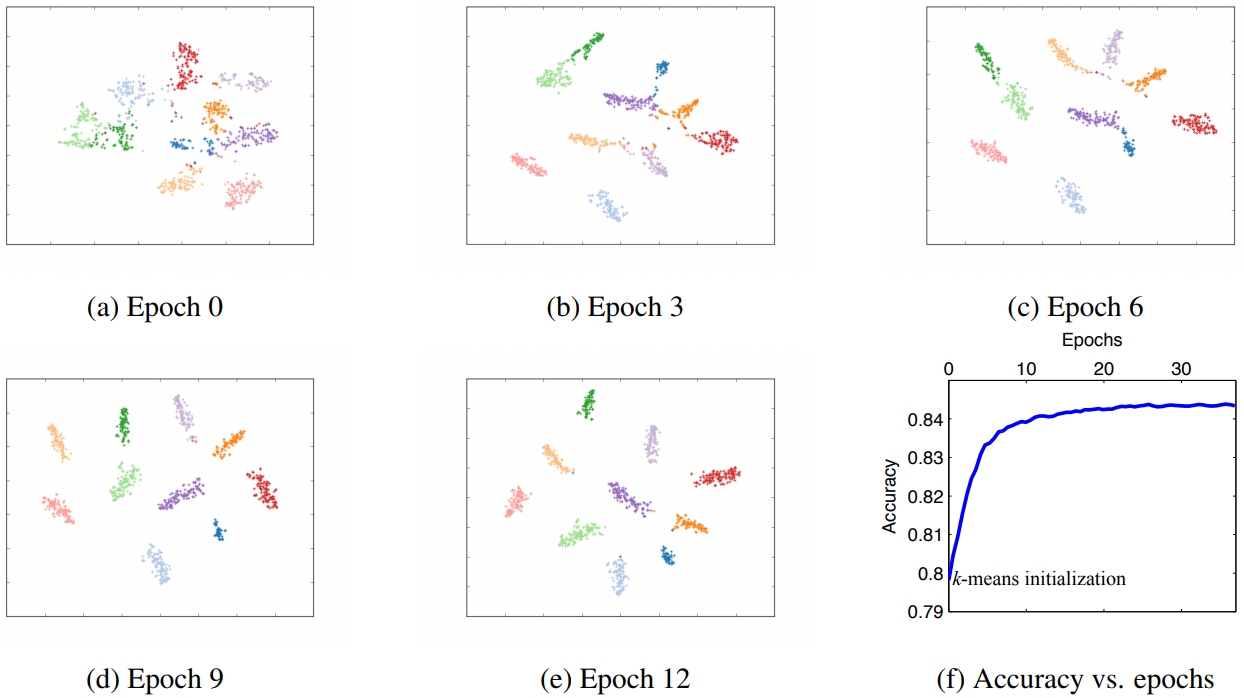# Unsupervised Deep Embedding for Clustering Analysis

## WHY?

There had been little study on learning representation that focus on clustering.

## WHAT?

Deep Embedding Clustering(DEC) consists of two phases: parameter initialization with a deep autoencoder and (2) parameter optimization. This paper first describe the second phase. Assume encoder and inital cluster centroids are given, two steps are alternated to improve clustering: 1) compute soft assignment of embedded points to centroids, and 2) update encoder and refine centroids.Student’s t-distriution is used as kernel for soft assignment. An auxiliary distribution p is designed to help learn from high confidence assignments. KL-divergence between soft assignment and auxiliary distribution is minimize by updating encoder and centroids. q_{ij} = \frac{(1+\|z_i - \mu_j\|^2/\alpha)^{-\frac{\alpha+1}{2}}}{\sum_{j'}(1+\|z_i - \mu_j\|^2/\alpha)^{-\frac{\alpha+1}{2}}}\\ p_{ij} = \frac{q_{ij}^2/f_j}{\sum_{j'}q_{ij'}^2/f_{j'}}, f_j = \sum_i q_{ij}\\ L = KL(P\|Q) = \sum_i \sum_j p_ij \log \frac{p_{ij}}{q_{ij}}$q_{ij} = \frac{(1+\|z_i - \mu_j\|^2/\alpha)^{-\frac{\alpha+1}{2}}}{\sum_{j'}(1+\|z_i - \mu_j\|^2/\alpha)^{-\frac{\alpha+1}{2}}}\\ p_{ij} = \frac{q_{ij}^2/f_j}{\sum_{j'}q_{ij'}^2/f_{j'}}, f_j = \sum_i q_{ij}\\ L = KL(P\|Q) = \sum_i \sum_j p_ij \log \frac{p_{ij}}{q_{ij}}$ Trained Stacked Autoencoder(SAE) with denoising autoencoder for each layer is used to initialize the embeddings. Initial centroids are determined by k-means clustering of initial embeddings.

## So?

DEC outperformed many clustering methods including k-means, LDMGI, and SEC in unsupervised clustering task of MNIST, STL-10, and Reuters.Visualization verified that embeddings are well separated.

## Critic

I like the idea to learn representation jointly with clustering, auxiliary target distribution seems like quite aritrary to me.

Powered by aiden